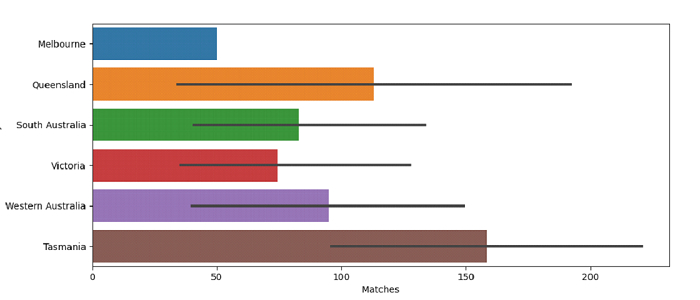# Python Pandas - Draw a set of horizontal bar plots with Seaborn

Bar Plot in Seaborn is used to show point estimates and confidence intervals as rectangular bars. The seaborn.barplot() is used for creating horizontal bar plots.

Let’s say the following is our dataset in the form of a CSV file − Cricketers2.csv

At first, import the required libraries −

import seaborn as sb
import pandas as pd
import matplotlib.pyplot as plt

Load data from a CSV file into a Pandas DataFrame −

dataFrame = pd.read_csv("C:\Users\amit_\Desktop\Cricketers2.csv")


Plotting horizontal bar plots −

sb.barplot(x = "Matches", y = "Academy", data= dataFrame)

## Example

Following is the code −

import seaborn as sb
import pandas as pd
import matplotlib.pyplot as plt

# Load data from a CSV file into a Pandas DataFrame

# plotting horizontal bar plots
sb.barplot(x = "Matches", y = "Academy", data= dataFrame)

# display
plt.show()

## Output

This will produce the following output −